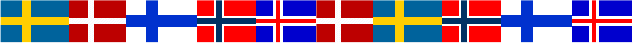www.gllamm.org

## 11 June 2003, Uppsala, Sweden

### Brief Description

Andrew Pickles, Sophia Rabe-Hesketh and Anders Skrondal will present a one-day course on Multilevel and latent variable modelling using gllamm on 11 June 2003 before the International Biometric Society Nordic Regional Conference 2003 in Uppsala, Sweden.

Multilevel models, or generalised linear mixed models are useful for longitudinal data, custer-randomised trials, surveys with cluster-sampling, genetic studies, meta-analysis and many other applications. The random coefficients in generalised linear mixed models can be interpreted as latent variables whose purpose is to model between-cluster variability and induce (or explain) within-cluster correlations. Latent variables are also often used to represent the true value of a variable measured with error, a typical example being diet (continuous latent variable) or diagnosis (categorical latent variable). Measurement models relating the measured variables to the latent variable can be estimated to investigate the characteristics of measurement instruments or diagnostic tests. Such measurement models can also form part of structural equation models relating latent variables to other latent or observed variables. An important application in biostatistics is regression with covariate measurement error. Finally, latent variables can be used to model the dependence between different processes, for instance the response of interest in a clinical trial and the (non-ignorable) drop-out process.

The course is structured in three parts: (1) generalised linear mixed models, (2) measurement models and (3) structural equation models. After introducing each kind of modelling, we will discuss examples, showing how the models can be estimated by maximum likelihood using the Stata program gllamm. We will also discuss prediction, including empirical Bayes methods for assigning values to latent variables, diagnostics and simulation using gllamm.

The course assumes a thorough knowledge of generalised linear models. Familiarity with Stata would be beneficial and experience with some command-based statistical package is essential.

### Provisional Course Outline

Time Topics
9.00-11.00 Generalised linear mixed models
• Random coefficient models
• Multilevel models
• Latent trajectory models
• Empirical Bayes and diagnostics
11.00-11.30 Break
11.30-13.00 Measurement models
• Factor models
• Item response models
• Latent class models
• Latent scoring and classification
13.00-14.00 Lunch
14.00-16.00 Structural equation models
• Covariate measurement error
• Continuous/categorical covariates
• Replicate measurements/validation samples
• Multivariate growth models
• Selection models for missing data
16.00-16.30 Break
16.30-17.30 Recent developments

### Registration

Registration will be possible from January 2003 via the Biometric Society Conference webpage